## Tutorial: Stream & catchment delineation using GRASS tools

### 3. Calculate flow accumulation and flow direction

The next step is to calculate the accumulation of water over the filled DEM.

1. In the Processing Toolbox choose GRASS | Raster (r.*) | r.watershed.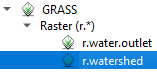The r.watershed tool has a lot of settings. Check the GRASS manual page for this tool to learn more about the settings of the algorithms.

2. In the r.watershed dialogue choose DEM_filled as Elevation. Set the Minimum size of exterior watershed basin to 500 pixels and check the box to Enable Single Flow Direction (D8) flow. Save the Number of cells that drain through each cell to accumulation.tif and save the Drainage direction to flowdir.tif. Uncheck the other outputs, we don't need them.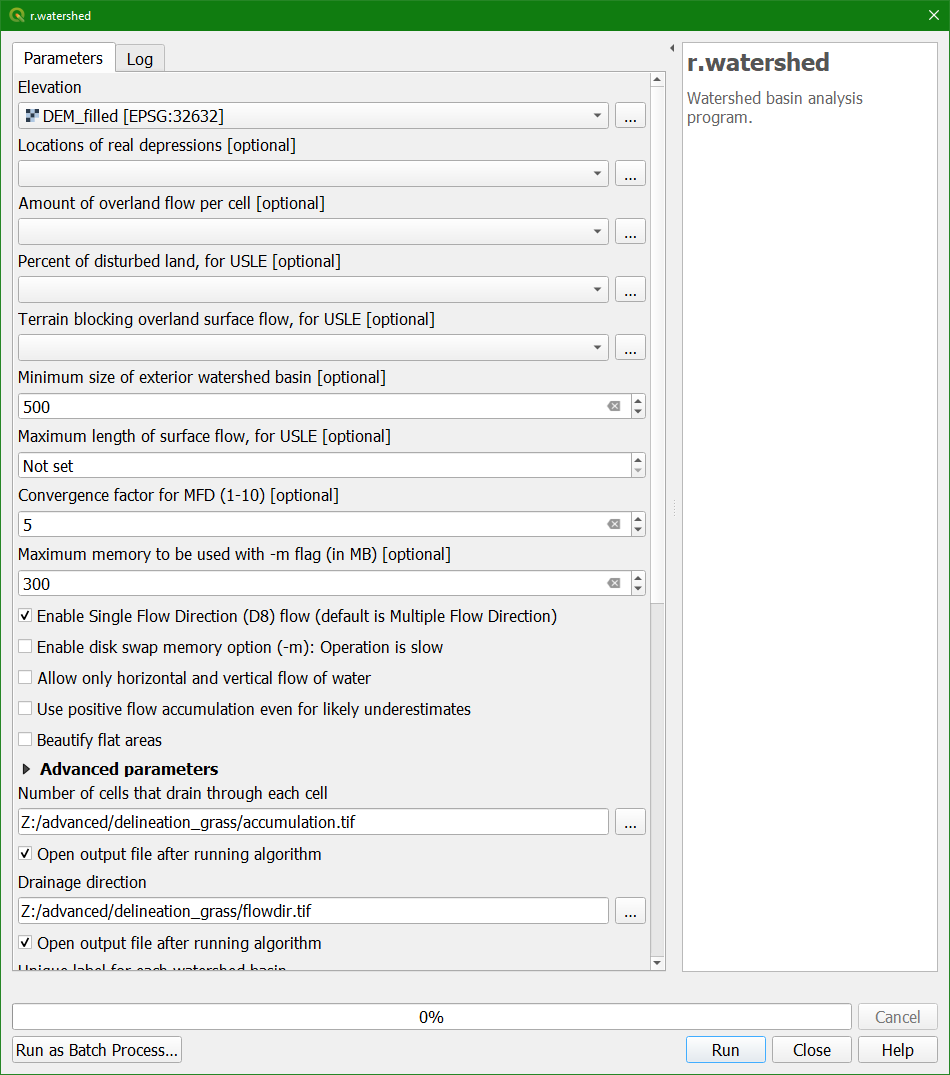3. Click Run. Close the dialogue after processing. Ignore the warnings in red.

The flow direction layer is encoded using the GRASS definition: Drainage is 8 directions numbered counter-clockwise starting from 1 in north-east direction. The value 0 indicates that the cell is a depression area. Negative values indicate that surface runoff is leaving the boundaries of the current geographic region. The absolute value of these negative cells indicates the direction of flow. Therefore we need to convert the flowdir layer to absolute values.

4. In the main menu go to Raster | Raster Calculator.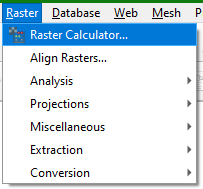5. In the Raster Calculator compose the following equation:

 ( "flowdir@1" < 0 ) * -1 * "flowdir@1" + ( "flowdir@1" >= 0 ) * "flowdir@1"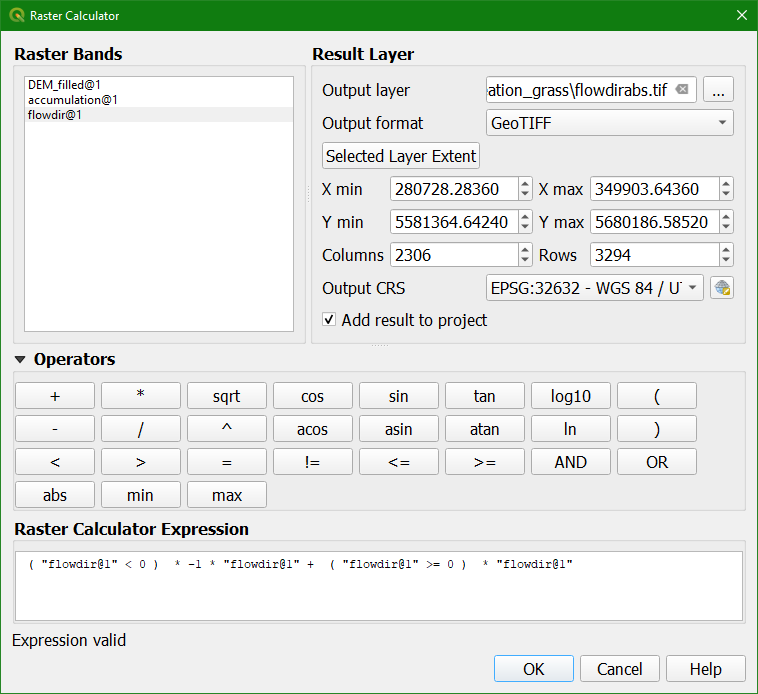This equation is a condition which reads as: if the flow direction values are less than zero then the result is boolean True (1), else boolean False (0). Multiply this with -1 and the flow direction . In this way the first part of the equation results in the absolute values of the negative flow directions. Then we add the condition if the cells are larger than or equal to zero, give boolean True (1), else boolean False (0) and multiply this with the flow direction values. This means that non-negative flow direction values will keep their original value.

6. Save the result as flowdirabs.tif and click OK.

Normally we would style the flow direction result with a directional grid, but that's not part of this tutorial.

7. Remove the original flowdir layer from the Layers panel.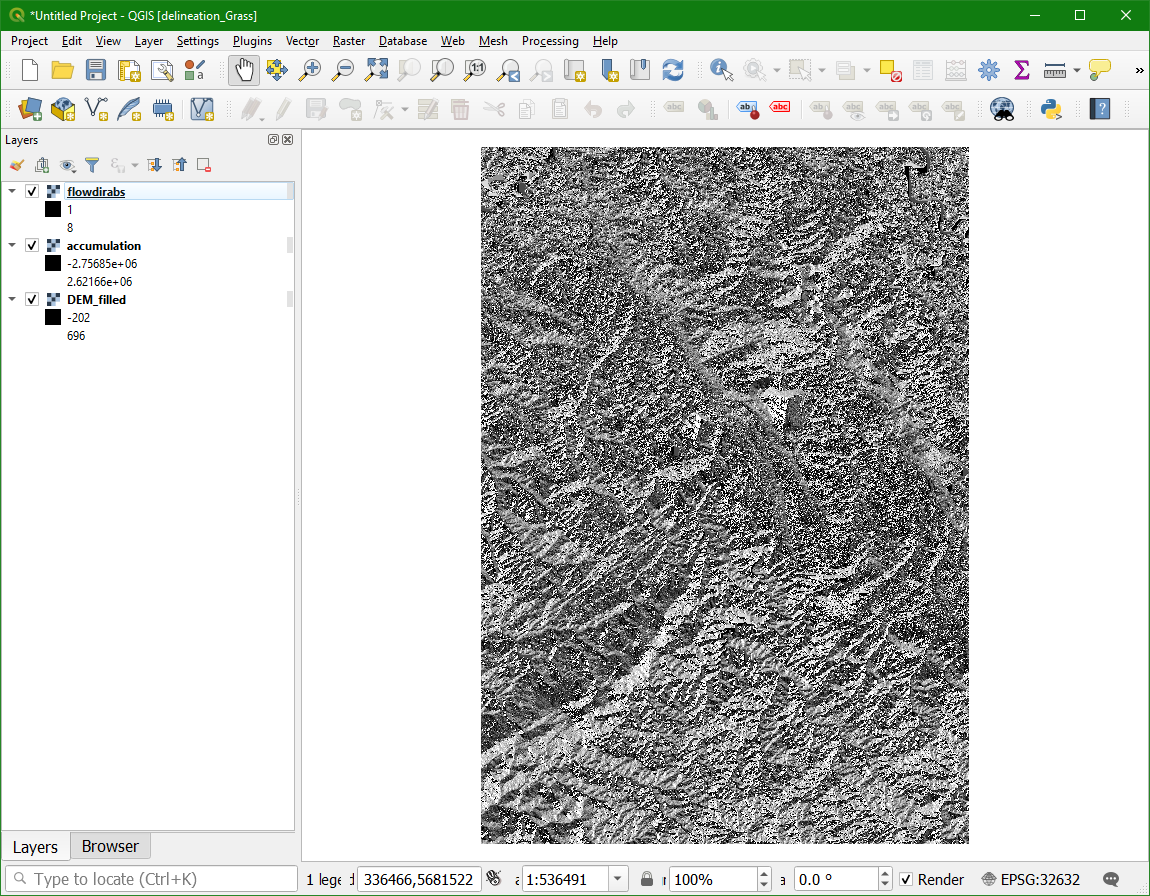The next step is to determine at which flow accumulation we can consider pixels as part of a channel.